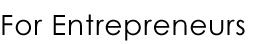June 4, 2020# Acid-Test Ratio# Definition of Acid-Test Ratio

The Acid-Test Ratio is calculated by subtracting the inventory from the current assets and then dividing that result by current liabilities.
(Definition continues below)

By looking at the acid-test ratio, or the acid ratio as some refer to it, you can get a quick snapshot of the health of a business.

Acid-Test Ratio References On This Site

These Gaebler.com articles mention this glossary term:

• Liquidity Ratios - Also referred to as an acid-test ratio, a quick ratio differs from a current ratio in...
• Liquidity Ratios - Also referred to as an acid-test ratio, a quick ratio differs from a current ratio in...